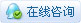# 自带保护色的变色龙灯

 变色龙LED灯的粗略效果预览↓↓↓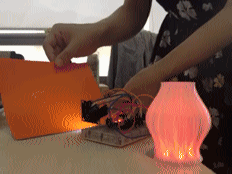【Intro】     变色龙是一种善变的树栖爬行类动物，为了逃避天敌的侵犯和接近自己的猎物，它们常在不经意间改变身体颜色，从而让自己融入周围的环境之中。    由于受到这种拉风变色技能的刺激，自己也想做一个会变色的小东西，可以根据周围事物不同的颜色来改变自己的颜色。当然，与变色龙的变色技能来自于皮肤三层色素细胞不同的是，这里想要实现变色功能就要用到一个颜色传感器和一个LED加上一个控制器和若干导线即可。     接下来，还需要一个容器来装这些元件，有条件的话可以根据自己喜好制作一个容器。这里我选用了一个使用较透明的材料通过3D打印的小灯罩作为这个会变色LED灯的容器。 【硬件列表】 【步骤1】准备好需要的元器件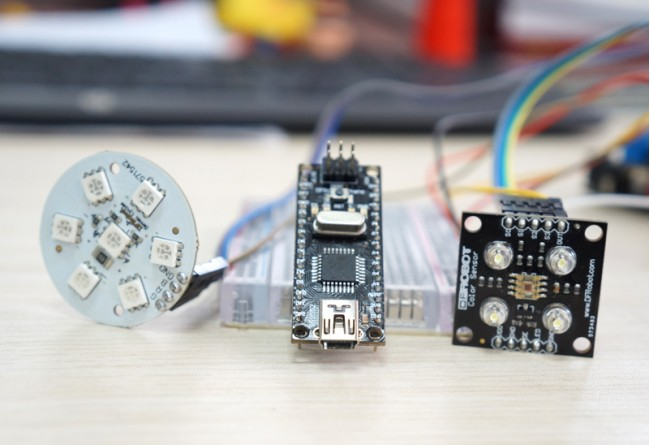因为容器的空间可能比较有限，如需要达到最佳的效果的话，尽量选用体积小一点的控制器。本人选用了Nano控制器，该控制器最大的特点就是体积小、功能强、低功耗可使用电池供电。 【步骤2】连接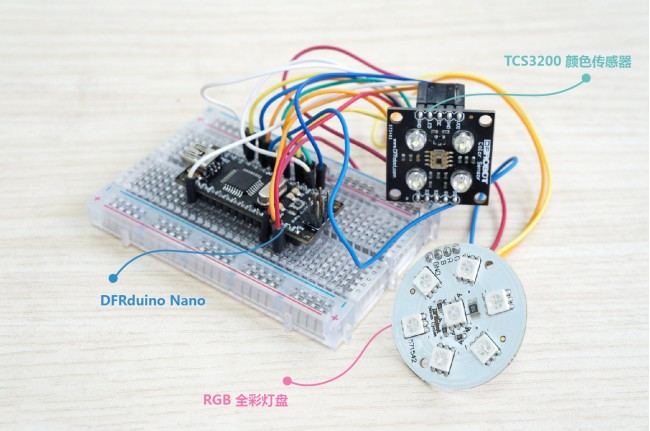将颜色传感器与Nano控制器用导线连接起来，这里注意每个端口的连接要结合软件定义正确连接（传感器的LED端接Nano的3.3V端）由于Nano的端口有限，所以扩展几个需要的端口，如下图所示：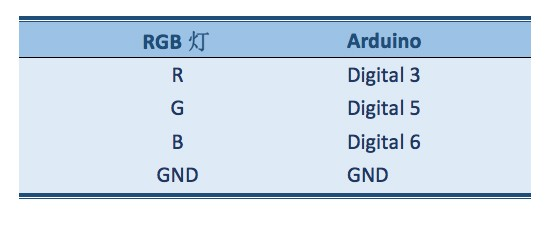下图所示为一个使用DFRuino UNO 以及 IO传感器扩展板的版本：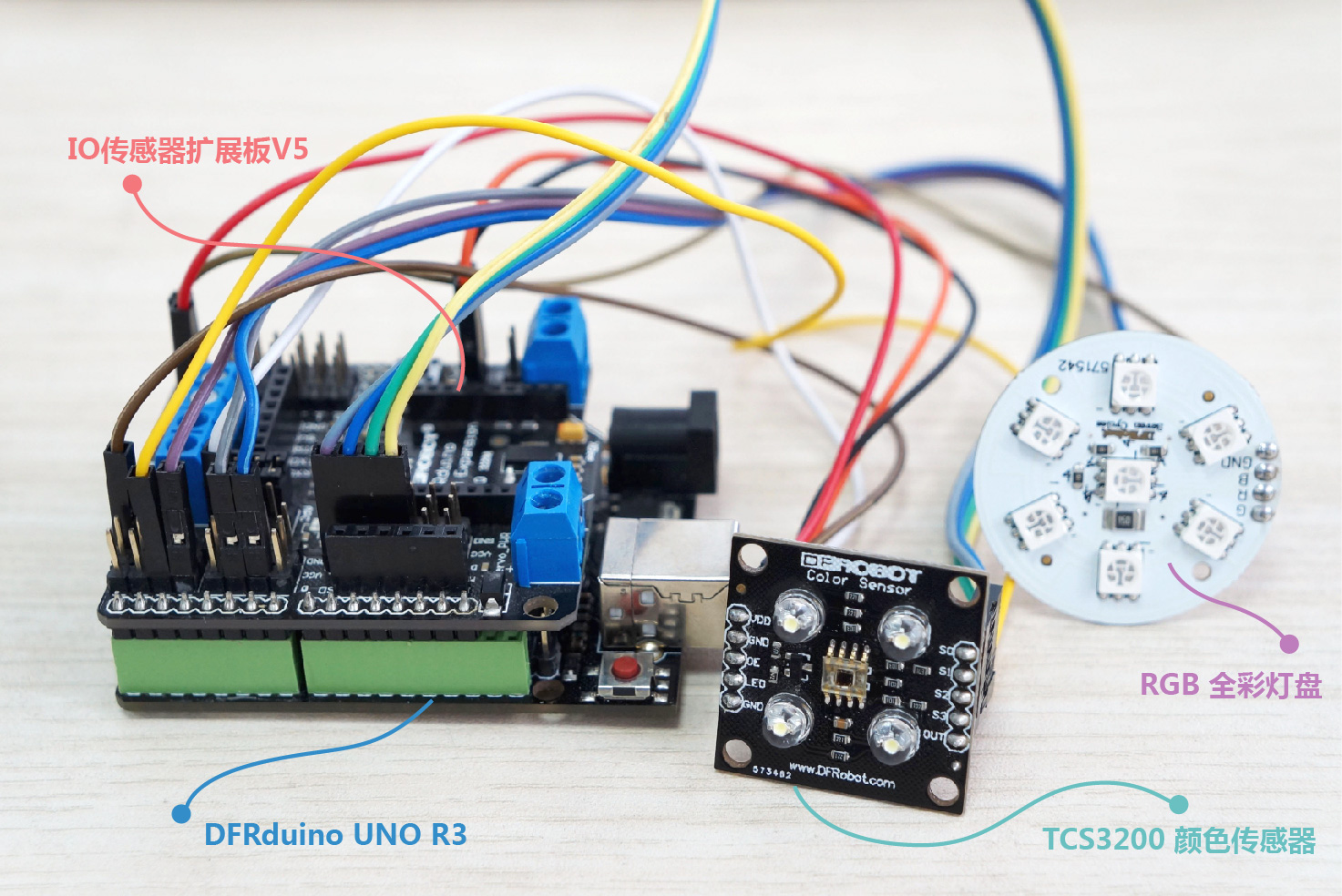【步骤3】下载程序 所有元件连接好后将编写好的代码烧到Nano控制器中代码中需要使用到Metro库，需要先安装。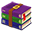Metro.zip (79.7 KB, 下载次数: 839) 2014-1-22 16:04 上传 点击文件名下载附件 Metro库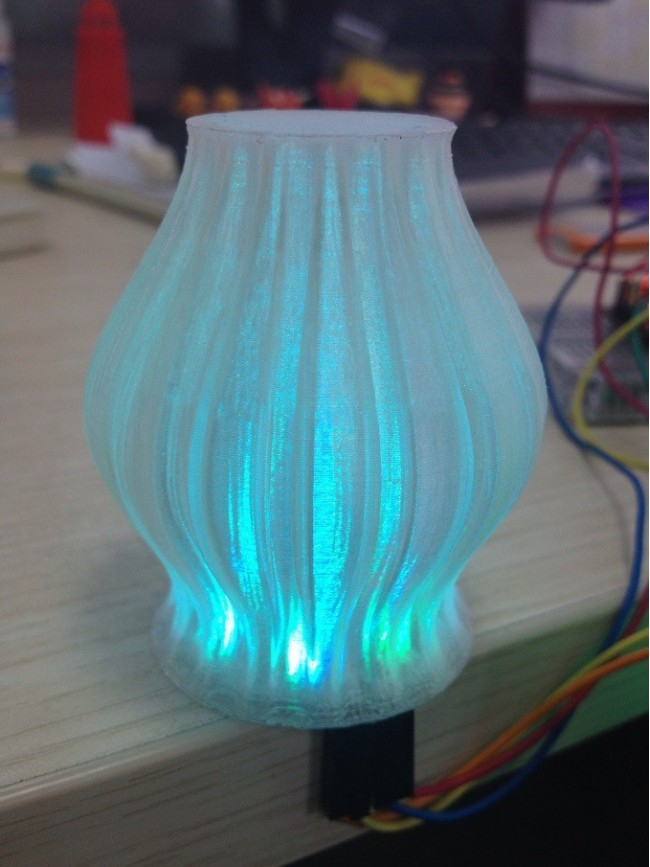注意：将不同颜色纸片放到传感器下，盒子就会发出相应颜色的光。由于环境光可能会对传感器采集的数据有一定影响，所以在晚上测试效果应该会更好。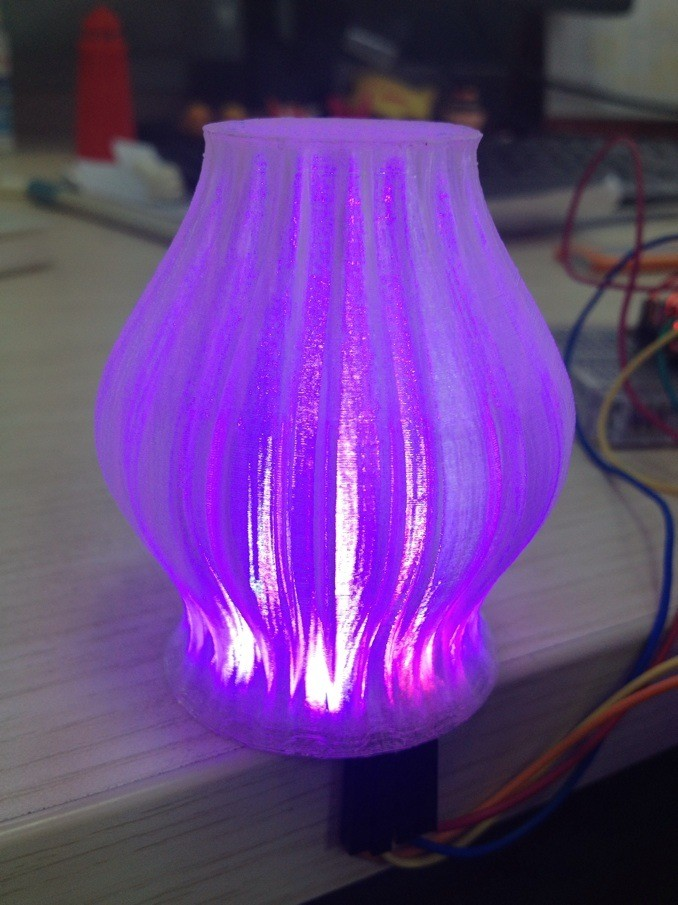【代码】 [size=16.363636016845703px]#include   // 函数库 #include Metro ledlightup = Metro(60,true); Metro processdata = Metro(50,true); Metro TcsTrigger = Metro(10,true); int s0=8,s1=9,s2=10,s3=11; // 颜色传感器端口定义 int out=2; int flag=0;  //变量初始化 int counter=0; int countR=0,countG=0,countB=0; void setup() {   Serial.begin(115200);   pinMode(s0,OUTPUT);   pinMode(s1,OUTPUT);   pinMode(s2,OUTPUT);   pinMode(s3,OUTPUT);   analogWrite(3,0);   analogWrite(5,0);   analogWrite(6,0); } void TCS() {   digitalWrite(s0,HIGH);   digitalWrite(s1,HIGH);   attachInterrupt(0, ISR_INTO, CHANGE); } void ISR_INTO() {   counter++; } int Raverage = 0; int Baverage = 0; int Gaverage = 0; void loop()       //   函数调用 {   TCS();   if(TcsTrigger.check()){     Tcstrigger();   }   if(processdata.check()){     procedata();   }      if(ledlightup.check()){    ledup();   } } void Tcstrigger()  //   数据采集 {       flag++;     if(flag==1){       digitalWrite(s2,LOW);       digitalWrite(s3,LOW);          countR=counter;            digitalWrite(s2,HIGH);       digitalWrite(s3,HIGH);     }          else if(flag==2){       countG=counter;             digitalWrite(s2,LOW);       digitalWrite(s3,HIGH);       }     else if(flag==3){       countB=counter;          digitalWrite(s2,LOW);       digitalWrite(s3,LOW);          flag=0;     }     counter=0; } void  procedata()        // 数据处理 {       static int Rinput ={       0,0,0,0,0                }     ,Binput ={       0,0,0,0,0                }     ,Ginput ={       0,0,0,0,0                };     for(int i = 4;i > 0;i--){       Rinput[i] = Rinput[i-1];       Binput[i] = Binput[i-1];       Ginput[i] = Ginput[i-1];     }        if(countR < 2500)       Rinput = countR;     else       Rinput = Rinput;     if(countB < 2500)       Binput = countB;     else       Binput = Binput;     if(countG < 2500)       Ginput = countG;     else       Ginput = Ginput;        Raverage = 0;     Baverage = 0;     Gaverage = 0;     for(int i = 0;i <= 4;i++){       Raverage += Rinput[i];       Baverage += Binput[i];       Gaverage += Ginput[i];     }     Raverage /= 5;     Baverage /= 5;     Gaverage /= 5;        } void  ledup()     //数据输出 {     int ledvalueR = Raverage;     int ledvalueG = Gaverage;     int ledvalueB = Baverage;     ledvalueR = constrain(ledvalueR,350,1700);     ledvalueB = constrain(ledvalueB,350,1500);     ledvalueG = constrain(ledvalueG,350,1650);      Serial.print("R:");     Serial.print(Raverage,DEC);     Serial.print(" ");     Serial.print(ledvalueR,DEC);     Serial.print("B:");     Serial.print(Baverage,DEC);     Serial.print(" ");     Serial.print(ledvalueB,DEC);     Serial.print("G:");     Serial.print(Gaverage,DEC);     Serial.print(" ");     Serial.println(ledvalueG,DEC);               ledvalueR = map(ledvalueR,350,1700,0,255);     ledvalueB = map(ledvalueB,350,1500,0,255);     ledvalueG = map(ledvalueG,350,1650,0,255);     analogWrite(3,ledvalueR);        analogWrite(6,ledvalueB);     analogWrite(5,ledvalueG);    }复制代码tutorials### lengyuexinghun学徒

 楼主，连接图怎么打不开啊### Ash管理员 楼主|

 lengyuexinghun 发表于 2014-3-13 11:24 楼主，连接图怎么打不开啊 谢谢提醒，重新上传了，应该没问题了。### lengyuexinghun学徒

 谢谢啦，我对这个变色龙很感兴趣，正准备做一个试试。### 爱国者一号初级技匠

 楼主你好，我是一个没有编程经验的人，按照我的思路，把程序直接烧进硬件就OK了，但前面你说代码需要Metro库，需下载，我不是很懂。楼主您能向我我讲解一下Metro库在烧录软件中的作用吗？有了您的讲解我将会进步。谢谢楼主### 再粗点再大点学徒

 学习了### lilei8488中级技师

 好的创意

 您需要登录后才可以回帖 登录 | 立即注册 本版积分规则 回帖并转播 回帖后跳转到最后一页